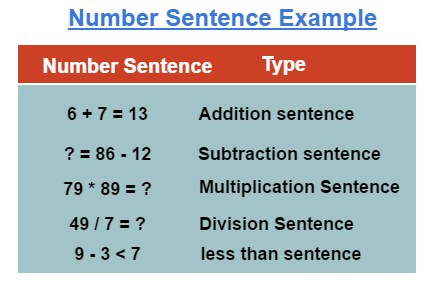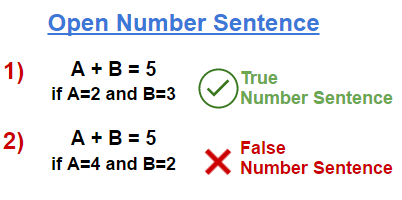# What is a Number Sentence? - Definition & Examples

## What is a Number Sentence?

A number sentence is a sequence of expression that contain numbers and symbols of operations like equal, greater than, less than, all arithmetic operations that give sometimes true result or sometimes false. So this is not necessary that any number sentence must be true. They are used in a way to record the computation process of solving a mathematical problem. Number sentence gives solution to the problems which basically involved numbers and the relationship between them that must be identified. Number sentence is just like a written sentence which is a string of words in maths we can say it is an expression that tells a fact.## Checking number sentence

Let’s define a numbers sentence like 2+2=4 so we put together two groups of item and result is 4. Now we examine what make this is a number sentence in that we are going to check elements of number sentence. Firstly we check that it has numbers, then for mathematical operations, then it should have equal sign numbers after it. Now we check for the few condition, that they are still number sentence or not. Let’s take a situation when there is no number in the expression then definitely not a number sentence because the operations performed on number only. Another one is let’s take out the addition sign between the both numbers, then again it is not.

## Open Number Sentence

Open number sentence is an expression that contain variables and result of which either become true or false depending on the value of the variable. Let’s take an example to understand the concept of Open number sentence. A + B = 5 the expression can be true or false that depend on the value of A and B if the value of A=2 and B=3 then the given expression is true and comes under True number sentence but if the value of A=4 and B=2 then the given expression is false number sentence.